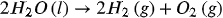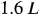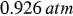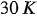1
256
views
42
Problem

# Problem 42

## Chapter 5: GasesTextbook ExpertVerified Tutor
25 Oct 2021

#### Given information

The balanced chemical equation for the given reaction is,The volume of oxygen gas formed in the reaction isThe pressure of oxygen gas isThe temperature of the reaction is#### Step-by-step explanation

Step 1.

Calculate the number of moles of oxygen gas in the given reaction by using the ideal gas equation.